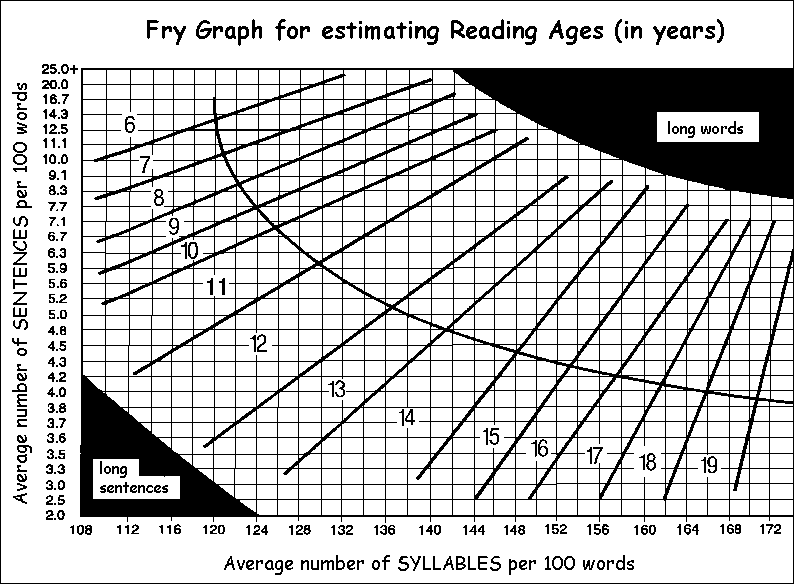# Readability of Texts

Sample text for determining readability index

Flesch-Kincaid Formula
This is a US Government Department of Defense standard test
(i) Calculate L, the average sentence length (number of words ÷ number of sentences).  Estimate the number of sentences to the nearest tenth,where necessary.
(ii) Calculate N, the average number of syllables per word (number of syllables ÷ number of words).
Then grade level = (L+N)x0.4
Reading Age = [(L+N)x0.4]+5 years

'SMOG' Formula
Select samples of 30 consecutive sentences.
In each sample, count the number of words with 3 or more syllables.
Find the average number, N.
Then grade level = (square root of N) + 3.
Reading Age = (square root of N) + 8 years.
This test tends to give higher values than the other formula, because it is intended to predict the level necessary for 100% comprehension of the text.